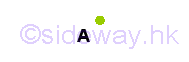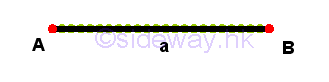output.to from Sideway
Geometry

Euclid's Elements

Geometric Element

Draft for Information Only

# Content

`  The Fundamentals of Geometric Figures    Dimensions     Elements of Geometric Figures`

## The Fundamentals of Geometric Figures

Geometry is the study of figures. There are many ways to describe a figure

### Dimensions

In general, a dimension is a phsical measurement of size, length, or distance in one direction. A dimension is also a descriptive measurement of the intrinsic property of an object.

### Elements of Geometric Figures

The fundamental notions of geometry.

1. A point is a primitive notion that has no dimensional attribute, 0-dimensional. A point can lie in a line, in a line, or in space.2. A line or straight line is a length notion that has one linear dimensional attribute called length, 1-dimensional. A line has no breadth, and thickness and can lie in a plane or in space. The extremities of a line are points.3. A plane, flat plane or Euclidean plane is an area notion that has two linear dimensional attributes called length and breadth, 2-dimensional. A plane has no thickness and can lie in space. The extremities of a plane are lines and the extremities of a line are points. Therse points are called vertices.4. A space, cuboid space or Euclidean space is an volume notion that has three linear dimensional attributes called length, breadth, and thickness, 3-dimensional. The extremities of a plane are lines and the extremities of a line are points. The extremities of a space are planes, the extremities of a plane are lines and the extremities of a line are points. Therse points are called vertices.

The fundamental objects of geometry for describing geometric figures.

1. A vertice is a 0-dimensional object but a vertice can lie in higher dimensional. A vertice itself has no length, breadth, and thickness.

2. A curve is a 1-dimensional object but a curve can lie in higher dimensional. A curve itself has no breadth and thickness.

3. A surface is a 2-dimensional object but a surface can lie in higher dimensional. A surface itself has no thickness.

4. A solid is a 3-dimensional object and a solid can lie in space only.

The fundamental elements of geometric figures.

1. A two dimensional figure, also called plane figure or planar figure, is a 2-dimensional object lying in a single plane but a planar figure can lie in higher dimensional.

A planar figure is a set of line segments and curve segments, which are also called sides and arcs. The sides and arcs of a two dimensional figure are called the edges of the figure. The edges themselves are one-dimensional object, but the edges lie in the two-dimensional plane forming a two dimensional figure. The interections of the edges are called the vertices or corners. Usually a vertex is refered to the end point of at least two edges, sometime a vertex can also be used to name the end point of the loose end of an edge. These vertices or points are zero-dimensional, but points lie in a one dimensional line in the two-dimensional plane.

2. A three dimensuon figure, also called solid figure, is a 3-dimensional object lying in three dmensional space only.

A solid figure is a set of plane regions and surface regions, which are also called surfaces. The surfaces of a three dimensional figure are called the faces of the figure. The face themselves are two-dimensional object, but the faces lie in the three dimensional space forming a three dimensional figure. The sides and arcs that are the edges of the faces of the solid figure are called the edges of the figure. The edges themselves are one-dimensional object, but the edges lie in the two-dimensional space forming a two dimensional figure in three dimensional space. Similarly, the interections of the edges are called the vertices or corners of the solid figure. These vertices are zero-dimensional, but points lie in the two-dimensional plane in three dimensional space.

©sidewayReferences

1. Hilbert, D. (translated by Townsend E.J.), 1902, The Foundations of Geometry, The Open Court Publishing Company, Chicago
2. Moore, E.H., 1902, On the projective axioms of geometry, Transactions of the American Mathematical Society 3: 142-158
3. Fitzpatrick R. (translated), Heiberg J.L. (Greek Text), Euclid (Author), 2008, Euclid's Elements of Geometry, (Richard Fitzpatrick)ID: 150200007 Last Updated: 2015/2/10 Revision: Ref:Home (5)

Business

Management

HBR (3)

Information

Recreation

Hobbies (7)

Culture

Chinese (1097)

English (336)

Reference (66)

Computer

Hardware (149)

Software

Application (187)

Digitization (24)

Numeric (19)

Programming

Web (644)CSS (SC)

ASP.NET (SC)

HTML

Knowledge Base

Common Color (SC)

Html 401 Special (SC)

OS (389)MS Windows

Windows10 (SC)

.NET Framework (SC)

DeskTop (7)

Knowledge

Mathematics

Formulas (8)

Number Theory (206)

Algebra (20)

Trigonometry (18)

Geometry (18)

Calculus (67)

Complex Analysis (21)

Engineering

Tables (8)

Mechanical

Mechanics (1)

Rigid Bodies

Statics (92)

Dynamics (37)

Fluid (5)

Control

Acoustics (19)

Biology (1)

Geography (1)

Latest Updated Links

Copyright © 2000-2019 Sideway . All rights reserved Disclaimers last modified on 10 Feb 2019